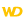WINDEV, WEBDEV AND WINDEV MOBILEONLINE HELPVersion: 15161718192021222324
 Home |Sign in | EnglishHelpWLanguageWLanguage syntaxType of dataAdvanced typesRealsType of realsNotesSee alsoCharacter stringsConverting C typesCurrenciesDateDateTimeDurationJSONNumericOperations available for the dates, times and durations, ...RealsRectangleThe "Buffer" typeThe Variant typeThe different types of dataThe different types of integersTimeUUID
• Type of reals
• Notes
• Default value
• Binary coding of reals
• Thousand separator
• Problems of precision with the reals
• Differences with WINDEV/WEBDEV 5.5
WINDEVWEBDEVWINDEV MobileOthersType of reals
Two types of reals are available in WLanguage:
 Real 8-byte realA real can contain up to 15 significant digits. The precision of decimals is not guaranteed. To perform precise calculations, use the "Currency" type.Value of a real:Minimum value: 1.7*10-308Maximum value: 1.7*10+308 4-byte real 4-byte realA 4-byte real can contain up to 6 significant digits. The precision of decimals is not guaranteed. To perform precise calculations, use the "Currency" type.Value of a 4-byte real:Minimum value: 3.4*10-38Maximum value: 3.4*10+38This type is not supported. It is replaced by a real.
Notes

Default value

A "Real" variable that is declared but not initialized is equal to 0.

Binary coding of reals

All the reals contain the same number of significant digits (15).
If the result of a calculation performed on reals involves more than 15 significant digits, this result will be automatically rounded to 15 significant digits. For a better precision, use the Currency or Numeric type.Versions 23 and later

Thousand separator

The 'Space' character or the '_' character can be used as thousand separator in the numbers. For example:
num1 is real
num1 = 123 456.478

num2 is real
num2 = 7_014.478New in version 23

Thousand separator

The 'Space' character or the '_' character can be used as thousand separator in the numbers. For example:
num1 is real
num1 = 123 456.478

num2 is real
num2 = 7_014.478

Thousand separator

The 'Space' character or the '_' character can be used as thousand separator in the numbers. For example:
num1 is real
num1 = 123 456.478

num2 is real
num2 = 7_014.478

Problems of precision with the reals

The operations performed with the "real" types are not precise because of the computing representation of reals.
Two reals that are equal mathematically speaking are not necessarily equal computer-wise and the ">", "<" or "=" operators can return results that are "false" mathematically speaking.
To fix some of these problems:
• the "=" operator is accurate to 10e-6 on the reals. Therefore, for two reals that are relatively close, the "=" and ">" operators (or "=" and "<") can return True for the same values.
• the conversion of real values into string uses a complex algorithm. This is why, in most cases, the display by trace is correct.
To avoid these problems, you must use the Currency or Numeric type that is using an exact memory representation.Differences with WINDEV/WEBDEV 5.5

The types of reals have been modified between WINDEV/WEBDEV 5.5 and WINDEV/WEBDEV 24:
Type of reals in version 5.5 Type of reals in version 24 Real 4-byte real Turbo real Not available anymore Double real Real
By default, in WINDEV or WEBDEV 5.5, the reals were coded on 4-byte reals. By default, in WINDEV or WEBDEV 24, the reals are coded on 8-bytes.
Minimum version required
• Version 9